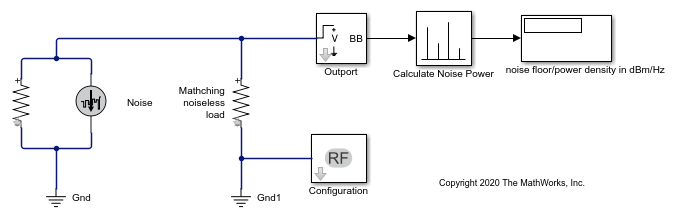# Explicitly Simulate Resistor Thermal Noise

Use the `Noise` block to calculate the classic thermal noise floor, `kT`, for a matched resistor circuit. Model configuration is as follows:

• Time step of the model is 1e-6 and frequency is 2 GHz.

• The `Resistor` noise source is modelled explicitly to make it noiseless. The resistance is 50 ohms. In the Resistor blocks, Simulate Noise is not selected.

• `Noise` current source parallel to the `Resistor` block models the noise. In the `Noise` block, the Source type is set to Ideal current to make it a current source. The Noise spectral density is defined as (4kT/R)(A2/Hz). The value of `k` is * The Masked block, Calculate Noise Power, calculates the noise floor as a standard deviation of the output signal.

```open_system('model_simrf_noise_source1') ```To run the model, select Simulation > Run. With the bandwidth included using the Configuration block, noise power is in the range of -173.98 to 174.1 dBm